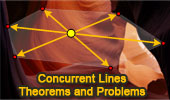# Geometry: Concurrent Lines, Theorems and Problems

## Concurrent Lines - Table of Content - Page 4Proposed Problem 138. Nagel's Theorem, Orthic Triangle, Concurrent Altitudes, Circumradius, Perpendicular.

Proposed Problem 137. Orthic Triangle, Concurrent Altitudes, Perpendicular, Incircle, Collinear Points, Parallelogram.

Proposed Problem 136. Orthic Triangle, Concurrent Altitudes, Perpendicular, Concyclic Points.

Proposed Problem 135. Orthic Triangle, Concurrent Altitudes, Perpendicular, Parallel

Proposed Problem 134. Orthic Triangle, Concurrent Altitudes, Angle Bisectors, Orthocenter, Incenter.

Proposed Problem 131. Van Aubel Theorem, Concurrent Cevians, Sum of Ratios.

Proposed Problem 130. Triangle, Concurrent Cevians, Sum of Ratios.

Proposed Problem 129. Triangle, Concurrent Cevians, Sum of Ratios.

Proposed Problem 128. Incenter of a Triangle, Concurrent Angle Bisectors, Sum of Ratios.

Go to page: Previous | 1 | 2 | 3 | 4 | 5 | Next